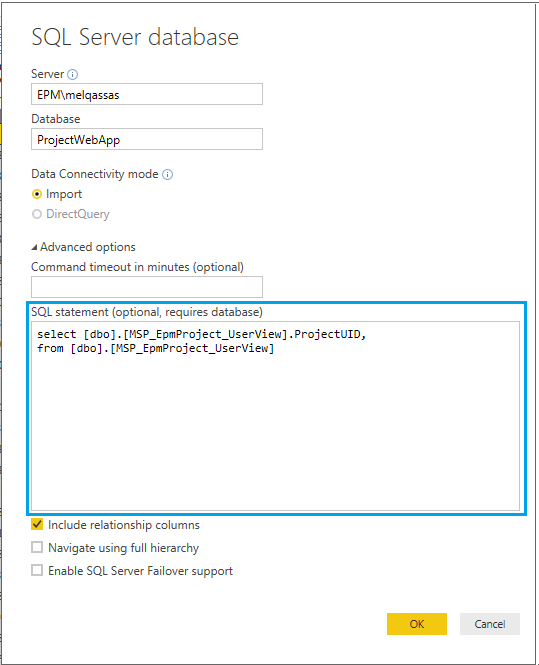# Convert SQL Query to DAX Formula in Power BI

0 like 0 dislike
14.7k views
in Power BI

I am new in Power BI, I was working in SSRS that I can use directly SQL query, I am planning to move from SSRS to Power BI now

Is there any tool to convert SQL query to DAX formula in Power BI?

2 like 0 dislike
by 64 137 299
selected by

## Power BI: Convert SQL Query to DAX Formula tool

Actually, there is NO such tool to Convert SQL Query to DAX Formula in Power BI. This is because DAX operates in a very different way from SQL Query.

### What is DAX?

• DAX stands for Data Analysis Expressions, and it's a formula language used throughout Power BI, Power Pivot, and SSAS Tabular.-
• DAX is a collection of functions, operators, and constants that can be used in a formula to create new information from the current data.

### What's SQL?

• SQL stands for Structured Query Language.
• It's used to perform a query on the database like SELECT, UPDATE, DELETE, INSERT, CREATE, DROP ..etc

### Examples to convert SQL Query to DAX formula in Power BI

Consider you need to get the count of `userID` from `User` table.

SQL Query

``````Select count(userID) from users
``````

The corresponding DAX formula in Power BI

``````Users Count = count('users'[userID])
``````

SQL Query

``````Select * from users
``````

The corresponding DAX formula in Power BI

``````Users = EVALUATE 'users'
``````

SQL Query

``````Select userID  from users
``````

The corresponding DAX formula in Power BI

``````Users = EVALUATE
SELECTCOLUMNS (
'users',
"userID", 'users'[userID]
)
``````

### How to convert complex SQL Query to DAX?

If you have a complex SQL query that has multiple subqueries, calculations, and many joins with different tables, so in this case, It's recommended to create a view in SQL and then read it in your Power BI dashboard directly!

• So when you `Get Date` in Power BI,• You can simply add your SQL query directly at `SQL statement` text box as shown below:This is would be helpful to avoid the double effort to maintain and convert complex SQL Query to DAX as well as it would improve the performance on the Power BI side because all the load and calculations will be done on the SQL side, not the Power BI side.